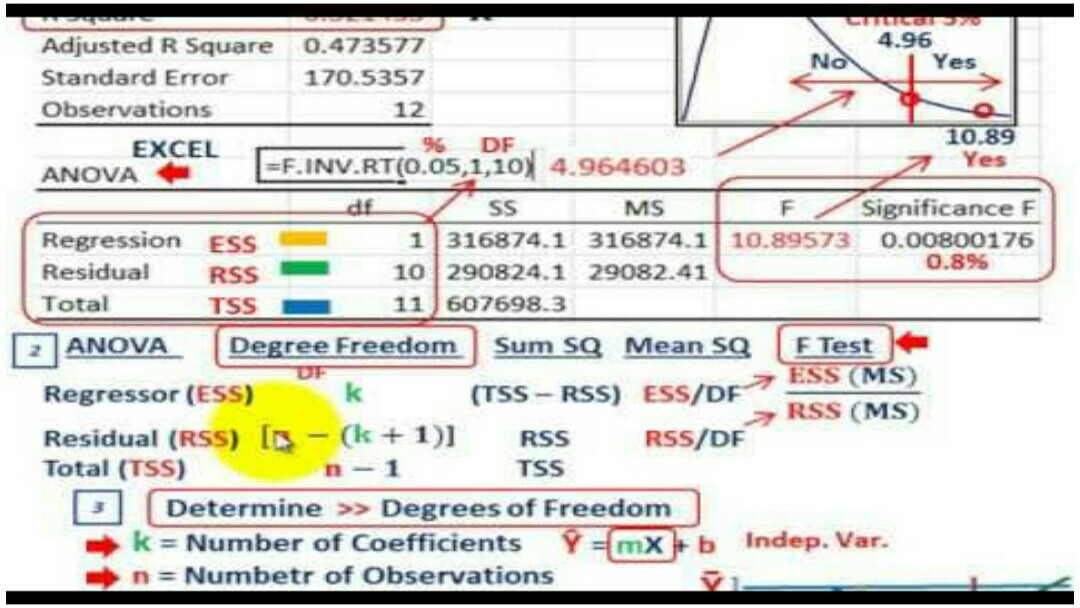-->## What is  Mean By Analysis of Variance (ANOVA)?

Analysis of variance (ANOVA) is an inferential statistics, the analysis tool used in statistics that splits an observed aggregate variability found inside a data set into two parts: systematic factors and random factors. The systematic factors have a statistical influence on the given data set, while the random factors do not. Analysts use the ANOVA test to determine the influence that independent variables have on the dependent variable in a regression study.

Some basic term used in analysis of variance are :- SST, SSE, SS treatment A, B ...K, MSE, and F calculated.